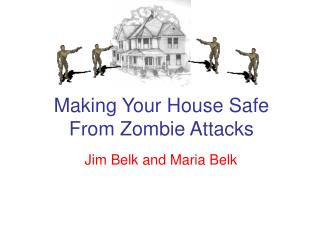# Making Your House Safe From Zombie Attacks - PowerPoint PPT PresentationDownload PresentationMaking Your House Safe From Zombie Attacks

Making Your House Safe From Zombie Attacks
Download Presentation## Making Your House Safe From Zombie Attacks

- - - - - - - - - - - - - - - - - - - - - - - - - - - E N D - - - - - - - - - - - - - - - - - - - - - - - - - - -
##### Presentation Transcript

1. Making Your House Safe From Zombie Attacks Jim Belk and Maria Belk

2. How can we construct a house so that we can escape from  grizzly bears? Let’s make this more precise.

3. Defining Grizzly Bear Graphs • We represent the house by a graph.

4. Defining Grizzly Bear Graphs • We represent the house by a graph. Vertices represent rooms.

5. Defining Grizzly Bear Graphs • We represent the house by a graph. Vertices represent rooms, and edges represent hallways.

6. Defining Grizzly Bear Graphs • We will allow loops and multiple edges in our graphs. • There is no exit from the house. • At the start of the game, you get to place yourself and the grizzly bears on the graph, wherever you want.

7. Defining Grizzly Bear Graphs • You move much, much faster than the grizzly bears.

8. Defining Zombie Graphs • You move much, much faster than the grizzly bears zombies.

9. Defining Zombie Graphs • You move much, much faster than the grizzly bears zombies. At the start of the game, you can set the speed of the zombies. • If you are ever in the same room as a zombie, or if two zombies are on either side of you in a hallway, you get eaten (and lose the game).

10. Defining Zombie Graphs • You know where all the zombies are at all times. • The zombie number of a graph is the minimum number of zombies needed to eventually catch and eat you assuming you use the best possible strategy.

11. Examples • A path has zombie number 1.

12. Examples • A tree has zombie number 1.

13. Examples • A cycle has zombie number 2. • Thus, a graph has zombie number 1 if and only if it is a tree.

14. Examples •  has zombie number 3. If only 2 zombies are on , you can always escape by moving towards an unoccupied vertex.

15. Examples •  has zombie number 3. If 3 zombies are on , you will be eaten. • In general,  has zombie number .

16. Cops and Robbers There is a similar well-known game: • A robber runs around a graph trying to escape cops, who travel by helicopter between adjacent vertices. The difference between the two games: • Zombies travel on edges. • Cops do not travel on edges. Instead they travel between adjacent vertices.

17. Cops and Robbers The zombie can catch the person: The cop cannot catch the robber:

18. Cop Number The cop number of a graph , denoted , is the minimum number of cops needed to eventually catch the robber, assuming the robber uses the best possible strategy. Theorem. (Seymour and Thomas) The cop number of a graph equals the treewidth plus 1. Theorem. The zombie number of a graph  is either  or .

19. Theorem. The zombie number of a graph  is either  or . The following graph has cop number 3 and zombie number 2:

20. Theorem. The zombie number of a graph  is either  or . If there are only 2 zombies, you can always move to whichever of the three vertices is the furthest from both zombies. The following graph has cop number 3 and zombie number 3.

21. Theorem. The zombie number of a graph  is either  or . A graph with cop number 3:

22. Theorem. The zombie number of a graph  is either  or . 3 zombies can catch you on this graph.

23. Theorem. The zombie number of a graph  is either  or . 3 zombies can catch you on this graph.

24. Theorem. The zombie number of a graph  is either  or . 3 zombies can catch you on this graph.

25. Theorem. The zombie number of a graph  is either  or . 3 zombies can catch you on this graph.

26. Forbidden Minors for Zombie number 2 Theorem. The “minimal” graphs with zombie number 3 are the following: A graph has zombie number 2 if does not contain one of the above graphs as a minor.

27. Further Questions • Which graphs have zombie number 3? • Zombie number 4? 5? 6? • If the cop number of the graph is known, how hard is it to determine the zombie number?

28. The End